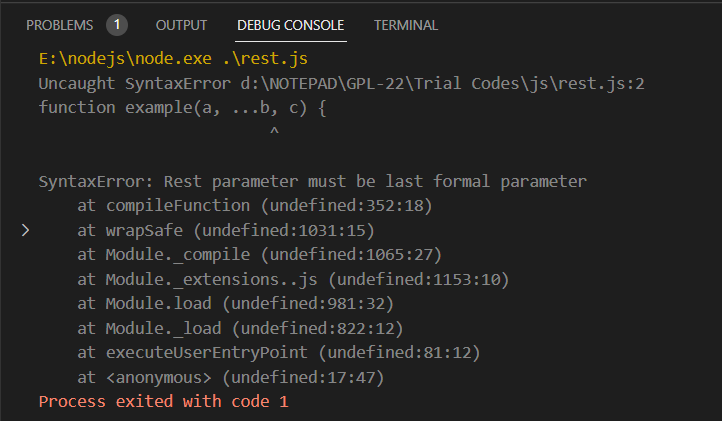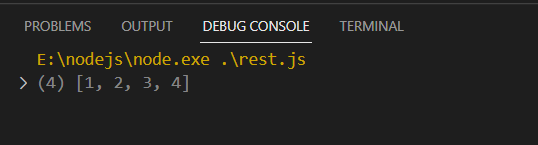GeeksforGeeks App
Open AppBrowser
Continue

# What happens if you do not use rest parameter as a last argument ?

The rest parameter (…) was introduced in ES6 to allow functions to accept an indefinite number of arguments, irrespective of how they are defined. This is useful when the number of parameters passed in a function call is not fixed.

Syntax:

```function f(arg1, arg2, arg3, ...arg4){
// body
}```

arg4 is the array formed using the rest parameter as defined above that stores all the remaining parameters which can further be used as required within the function. This makes it easier to write code more concisely and effectively.

The rest parameter is to be used keeping the following points in mind:

• There can only be one rest parameter in a function.
• It has to be the last argument in a function.

Note: In case the rest parameter is not used as the last argument, a SyntaxError is thrown stating that it has to be the last formal argument and the code is not executed.

The following examples depict the incorrect and the correct usage of the rest parameter respectively:-

Example 1: In this example, we are not using the rest parameter as the last argument.

## Javascript

 ``

Output:Explanation: In the above example, the function is defined using the rest parameter at a position other than the last, thus a SyntaxError is thrown as soon as the code is executed. This is because when a rest parameter is encountered in a function, all the parameters remaining are stored in the array formed by it, thus if it is used at a position other than the last then the arguments defined after the rest parameter are of no use.

Example 2: In this example, we are using the rest parameter as the last argument.

## Javascript

 ``

Output:Explanation: In the above example, the rest parameter is used as the last argument in the function definition, thus allowing it to collect all the remaining parameters into an array after the first two parameters. The function returns the array formed using the rest parameter and the same is printed on the console. Hence, the Rest parameter must be the last formal parameter in a function definition.

My Personal Notes arrow_drop_up